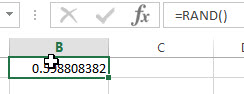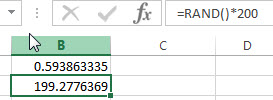# Excel RAND Function

This post will guide you how to use Excel RAND function with syntax and examples in Microsoft excel.

### Description

The Excel RAND function returns a random real number that is greater than or equal to 0 and less than 1. The RAND function is returned a new random real number when a worksheet is changed. So you can use this function to get a random number between 0 and 1.

The RAND function is a build-in function in Microsoft Excel and it is categorized as a Math and Trigonometry Function.

The RAND function is available in Excel 2016, Excel 2013, Excel 2010, Excel 2007, Excel 2003, Excel XP, Excel 2000, Excel 2011 for Mac.

### Syntax

The syntax of the RAND function is as below:

`=RAND ()`

The RAND function has no arguments.

Note:

• If you want to use RAND to generate a random number but don’t want the numbers to change every time the cell is calculated, you can enter =RAND() in the formula bar, and then press F9 to change the formula to a random number.

### Excel RAND Function Examples

The below examples will show you how to use Excel RAND Function to get a random number between 0 and 1.

1# get a random number greater than or equal to 0 and less than 1, enter the following formula in Cell B1.

=RAND()2# get a random number greater than or equal to 0 but less than 200, enter the following formula in Cell B2.

=RAND()*200### Related Functions

• Excel RANDBETWEEN function
The Excel RANDBETWEEN function returns a random integer number that is between the numbers you specify. The syntax of the RAND function is as below:= RANDBETWEEN (bottom,top)…